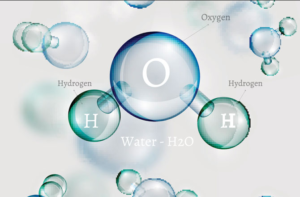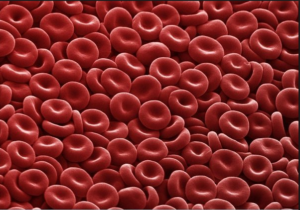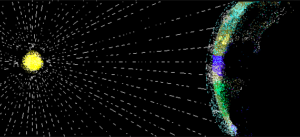## Problem Solving With Scientific Notation

### Learning Outcome

• Solve application problems involving scientific notationA water molecule.

## Solve Application Problems

Learning rules for exponents seems pointless without context, so let us explore some examples of using scientific notation that involve real problems. First, let us look at an example of how scientific notation can be used to describe real measurements.

Match each length in the table with the appropriate number of meters described in scientific notation below. Write your ideas in the textboxes provided before you look at the solution.

 The height of a desk Diameter of a water molecule Diameter of the sun at its equator Distance from Earth to Neptune Diameter of Earth at the equator Height of Mt. Everest (rounded) Diameter of an average human cell Diameter of a large grain of sand Distance a bullet travels in one second
 Power of 10, units in meters Length from table above $10^{12}$ $10^{9}$ $10^{6}$ $10^{4}$ $10^{2}$ $10^{0}$ $10^{-3}$ $10^{-5}$ $10^{-10}$Several red blood cells.

One of the most important parts of solving a “real-world” problem is translating the words into appropriate mathematical terms and recognizing when a well known formula may help. Here is an example that requires you to find the density of a cell given its mass and volume. Cells are not visible to the naked eye, so their measurements, as described with scientific notation, involve negative exponents.

### Example

Human cells come in a wide variety of shapes and sizes. The mass of an average human cell is about $2\times10^{-11}$ grams. Red blood cells are one of the smallest types of cells, clocking in at a volume of approximately $10^{-6}\text{ meters }^3$. Biologists have recently discovered how to use the density of some types of cells to indicate the presence of disorders such as sickle cell anemia or leukemia.  Density is calculated as $\frac{\text{ mass }}{\text{ volume }}$. Calculate the density of an average human cell.

The following video provides an example of how to find the number of operations a computer can perform in a very short amount of time.Light traveling from the sun to the earth.

In the next example, you will use another well known formula, $d=r\cdot{t}$, to find how long it takes light to travel from the sun to Earth. Unlike the previous example, the distance between the earth and the sun is massive, so the numbers you will work with have positive exponents.

### Example

The speed of light is $3\times10^{8}\frac{\text{ meters }}{\text{ second }}$. If the sun is $1.5\times10^{11}$ meters from earth, how many seconds does it take for sunlight to reach the earth?  Write your answer in scientific notation.

### Summary

Scientific notation was developed to assist mathematicians, scientists, and others when expressing and working with very large and very small numbers. Scientific notation follows a very specific format in which a number is expressed as the product of a number greater than or equal to one and less than ten times a power of $10$. The format is written $a\times10^{n}$, where $1\leq{a}<10$ and n is an integer. To multiply or divide numbers in scientific notation, you can use the commutative and associative properties to group the exponential terms together and apply the rules of exponents.

1. Orders of magnitude (mass). (n.d.). Retrieved May 26, 2016, from https://en.wikipedia.org/wiki/Orders_of_magnitude_(mass)
2. How Big is a Human Cell?
3. How big is a human cell? - Weizmann Institute of Science. (n.d.). Retrieved May $26, 2016$, from http://www.weizmann.ac.il/plants/Milo/images/humanCellSize120116Clean.pdf
4. Grover, W. H., Bryan, A. K., Diez-Silva, M., Suresh, S., Higgins, J. M., & Manalis, S. R. (2011). Measuring single-cell density. Proceedings of the National Academy of Sciences, 108(27), 10992-10996. doi:10.1073/pnas.1104651108